SCHOLATIC APTITUDE TEST 1998

MATHEMATICS

 Time: Two Hours(8.30 AM - 10.30 AM) Max. Marks: 60

NOTE:-

• Answers must be written in English or the medium of instruction of the candidate in High school.
• Attempt all questions.
• Answer all the questions in the booklets provided for the purpose. No pages should be removed from the booklets.
• There is no negative marking.
• Answer all questions of section I at one place. Same applies to section II. The remaining questions can be answered in any order.
• Answers to sections I and II must be supported by mathematical reasoning.
• Use of calculators, slide rule, graph paper and logarithmic, trigonometric and statistical tables is not permitted

Section I

This section has 5 questions. Each question is provided with five alternative answers. Only one of them is correct. Indicate the correct answer by Aor B or C or D or E. Order of the questions must be maintained. (5x2=10 Marks)

1. p is the smallest positive such that every positive integer greater than p can be written as a sum of two composite numbers. Then
A) p=3 B) p=6 C) p=10 D) p=11 E) None of these
2. ABC is a triangle such that ma > a/2, where ma = length of the median through A and a = length of BC. Then
 A) such a triangle does not exist B)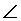A is acute C)A is obtuse D)A is right E) None of these
3. A vegetable shop keeps only four weights, one each of 1 kg, ½ kg, ¼ kg, 1/8 kg. A newly appointed shop assistant claims that he has so far taken once and only once each weighing possible with the available weights. If n is the number of all weighings possible and W the total weight of all possible weighings, then
 A) n=4,W=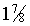kg B) n=16, W=30kg C) n=15,W=15 kg D) n=15, W=7.5 kg E) None of these
4.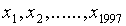are the roots of the polynomial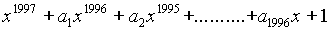and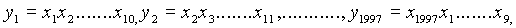Then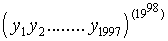is independent of
 A) All ai B) All ai, i ¹ C) None of ai D) All ai ,i even E) All ai ,i odd

5. Triangle ABC is isosceles, right angled at B and has area S. A circle is constructed with B as center and BA as radius. A semicircle is constructed externally on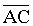, that is, on the side of AC which is opposite to that of B. Then the area of the crescent or sickle formed between the circle and the semicircle is
 A) 2S B) S/2 C) S D)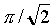S E) None of these

Section II

This section has 5 questions. Each question is in the form of a statement with a blank. Fill the blank so that the statement is true. Maintain the order of the questions. (5x2=10 Marks)

1. Numbers 1,2,3,…..,1998 are written in the natural order. Numbers in odd places are stricken off to obtain a new sequence. Numbers in odd places are stricken off from this sequence to obtain another sequence and so on, until only one term a is left. Then a=________
2. S is the circumcircle of an equilateral triangle ABC. A point D on S is such that C and D lie on opposite sides of AB. ThenADB=               radians
3.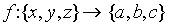is a bijection from a 3-element set into a 3-element set. It is given that exactly one of the following statements A,B,C is true and the remaining two are false: A: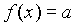B: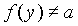C: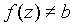. Then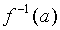=                     .
4. Given that x is real, the solution set of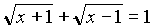is                       .
5. In the standard expansion of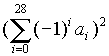, the number of terms appearing with -ve sign is                           .

Section III

This section has 5 questions. The solutions are short and methods, easily suggested. Very long and tedious solutions may not get full marks. (5x2=10 Marks)

1. Determine all positive integers n such that n+100 and n+168 are both perfect squares.
2. Determine with proof whether integers x and y can be found such that x+y and x2+y2 are consecutive integers.
3. The first term of an arithmetical progression is Log a and the second term is Log b. Express the sum to n terms as a logarithm.
4. p,q,r,s are positive real numbers. Prove that (p2+p+1)(q2+q+1)(r2+r+1)(s2+s+1) ³ 81pqrs
5. With the aid of a rough sketch describe how you will draw a direct common tangent to two circles having different radii. (No formal proof is required).

Section IV

This section has 6 questions. The solutions involve either slightly longer computations or subtler approaches. Even incomplete solutions may get partial marks. (6x5=30 Marks)

1. If x+y+z=0, then prove that
(x2+xy+y2)3 + (y2+yz+z2)3 + (z2+zx+x2)3 = 3(x2+xy+y2) (y2+yz+z2) (z2+zx+x2)
2. Simplify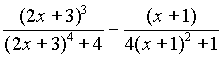till it is obtained equal to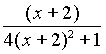3. a,b,c are distinct and p(x) is a polynomial in x, which leaves remainders a,b,c on division by x-a, x-b, x-c, respectively. Find the remainder obtained on division of p(x) by (x-a)(x-b)(x-c).
4. A point A is taken outside a circle of radius R. Two secants are drawn from this point: One passes through the center, the other at a distance of R/2 from the center. Find the area of the circular region enclosed between the two secants.
5. A right angled triangle has legs a,b, a>b. The right angle is bisected splitting the original triangle into two smaller triangles. Find the distance between the orthocenters of the smaller triangles using the co-ordinate geometry methods or otherwise.
6. If two sides and the enclosed median of a triangle are respectively equal to two sides and the enclosed median of another triangle, then prove that the two triangles are congruent.